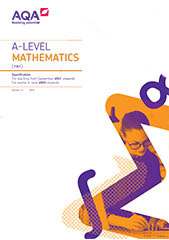# Specification at a glance

This qualification is linear. Linear means that students will sit all their exams at the end of the course.

## Subject content

Core content

• OT1: Mathematical argument, language and proof (page 11)
• OT2: Mathematical problem solving (page 11)
• OT3: Mathematical modelling (page 12)
• A: Proof (page 12)
• B: Algebra and functions (page 13)
• C: Coordinate geometry in the (x,y) plane (page 14)
• D: Sequences and series (page 15)
• E: Trigonometry (page 15)
• F: Exponentials and logarithms (page 17)
• G: Differentiation (page 18)
• H: Integration (page 19)
• I: Numerical methods (page 20)
• J: Vectors (page 20)
• K: Statistical sampling (page 21)
• L: Data presentation and interpretation (page 21)
• M: Probability (page 22)
• N: Statistical distributions (page 22)
• O: Statistical hypothesis testing (page 23)
• P: Quantities and units in mechanics (paqe 23)
• Q: Kinematics (page 23)
• R: Forces and Newton’s laws (page 24)
• S: Moments (page 25)

## Assessments

Paper 1

What's assessed

Any content from:
• A: Proof
• B: Algebra and functions
• C: Coordinate geometry
• D: Sequences and series
• E: Trigonometry
• F: Exponentials and logarithms
• G: Differentiation
• H: Integration
• I: Numerical methods

How it's assessed

• Written exam: 2 hours
• 100 marks
• 33⅓ % of A-level

Questions

A mix of question styles, from short, single-mark questions to multi-step problems.Paper 2

What's assessed

Any content from Paper 1 and content from:
• J: Vectors
• P: Quantities and units in mechanics
• Q: Kinematics
• R: Forces and Newton’s laws
• S: Moments

How it's assessed

• Written exam: 2 hours
• 100 marks
• 33⅓ % of A-level

Questions

A mix of question styles, from short, single-mark questions to multi-step problems.Paper 3

What's assessed

Any content from Paper 1 and content from:
• K: Statistical sampling
• L: Data presentation and Interpretation
• M: Probability
• N: Statistical distributions
• O: Statistical hypothesis testing

How it's assessed

• Written exam: 2 hours
• 100 marks
• 33⅓ % of A-level

Questions

A mix of question styles, from short, single-mark questions to multi-step problems.